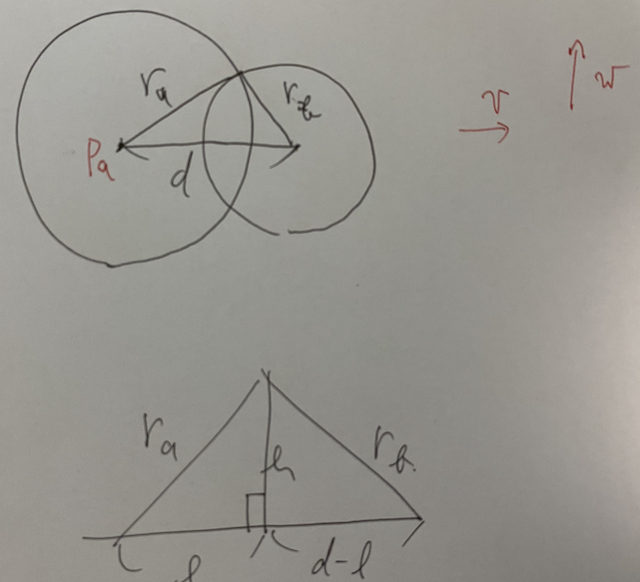# AtCoder Beginner Contest 157

Updated:

AtCoder Beginner Contest 157

• Review: 2020-06-18

# Solutions

## A - Duplex Printing

Flush $(N + 1) / 2$.

## B - Bingo

Do as they indicate us to. This is a little hard problem using for-loop.

## C - Guess The Number

We try all the possibilities for the integer $x$. We convert $x$ into string.

## D - Friend Suggestions

We use $u \sim _ F v$ and $u \sim _ B v$ to denote the friendship and the block-ship between $u$ and $v$, respectively. The friendship and the block-ship are not equivalence relationship, but we extend the friendship to the minimum equivalence relationship. We call it the friend-candidate-ship.

Fix the user $u$. Let $V _ u$ be the the equivalence class of the friend-candidate-ship. The set of the friend candidates of $u$ is $V _ u \setminus (\{ v \in V _ u \mid v \sim _ F v \} \cup \{ v \in V _ u \mid v \sim _ B v \}).$

We can calculate $V _ u$s in $O(N \log N)$-time by Union-Find with size-method. Then, we check whether $v \in V _ u$ or not for each $v$ who is a friend or a block user of $u$.

Note that each friend of $u$ is included in $V _ u$. We can omit the checks for friendship.

## E - Simple String Queries

### Solution #1

We can solve this problem by segment tree.

$tree[i][j] = 1$ if $S[j] = i$, otherwise $0$.

Initialization takes $O(N \log N)$. Each query is done by $O(\log N)$. Thus the total time complexity is $O(N \log N + Q \log N)$.

### Solution #2

We can solve this problem by the same idea using vector<set<int>> T(26);.

$T[c] =$ the set of indexes $ind$ with $S[ind] = c$.

Suppose that we answer to the query $[l, r]$. For $i = 0, \dots, 25$, we ask $T[i]$ the lower_bound of $l$. Let $it$ be that iterator. To check that there exists an element in $[l, r]$, we check *it <= r.

The total time complexity is $O(N \log N + Q \log N)$, too.

## F - Yakiniku Optimization Problem

### Observation

#### Binary search for $T$

The larger $T$ is, the greater the possibility is. Thus, this problem is solved by binary search for $T$. Hereinafter, we fix $T$ and we determine whether there exists a valid place for the heat source.

We estimate the lower bound and the upper bound. The former is $0$. The latter is $2 \times \max \{ \lvert x _ i \rvert, \lvert y _ i \rvert \} _ i \times \sqrt{2} \times \max c _ i \leq 1,000,000$. We set the initial bounds as these.

#### Condition to check

Let $p _ i = (x _ i, y _ i)$ be the place for the $i$-th meat. Let $H = (X, Y)$ be the place for the heat source. The condition is equivalent to the following.

• There exists at least $K$ indexes $i \in N$ so that $\lvert p _ i - H \rvert \leq T / c _ i$.

We can check this condition in $O(N)$-time.

#### Possible places for the heat source

Assume $N \geq 2$. Let $C _ i$ be the circle whose center is $p _ i$ and whose radius is $T / c _ i$. Let $D _ i$ the disk surrounded by $C _ i$. Assume that the condition holds. Then, we can assume that $D _ 0, \dots, D _ {K - 1}$ has the non-empty intersection and any $H \in \bigcap _ {i \in K} D _ i$ satisfies the condition.

We want to try finite possible points. What point should we check?

We fix two disks $D _ i$ and $D _ j$ with $i \neq j$ and $i, j \in K$. If $D _ i \subset D _ j$, it suffices to pick up the center of $D _ i$ and we can ignore $D _ j$. The similar holds if $D _ j \subset D _ i$. Otherwise, it suffices to pick up the points in $C _ i \cap C _ j$. They are at most two points.

To sum up these results, it suffices for us to try the following points.

• The centers $p _ i$ of the circle $C _ i$ for $i \in N$,
• The points $p \in C _ i \cap C _ j$ for $0 \leq i < j < N$.

They are at most $O(N ^ 2)$-points.

### Solution

What is left is to calculate the points of $C _ a \cap C _ b$. Let $p _ a, p _ b \in \mathbb{R} ^ 2$ the center of $C _ a, C _ b$, respectively. Let $r _ a, r _ b > 0$ the radius of $C _ a, C _ b$, respectively. Let $d = \lvert p _ b - p _ a \rvert$, $v = (p _ b - p _ a) / d$ and $w$ be the rotation of $v$ in $\pi / 2$.

Let $l$ and $h$ be as the following image. It holds that \begin{align} & & r _ a ^ 2 - l ^ 2 &= r _ b ^ 2 - (d - l) ^ 2, \ & \text{i.e.,} & l &= \frac{r _ a ^ 2 - r _ b ^ 2 + d ^ 2}{2d}. \end{align} Note that this is valid even if $l < 0$ or $l > d$.Let $t = r _ a ^ 2 - l ^ 2$. If $t < 0$, there exists no intersection point. We assume otherwise hereinafter. We see that $h = \sqrt{t}$. the two intersection points are as follows. $p _ a + lv \pm hw.$

Tags:

Categories: